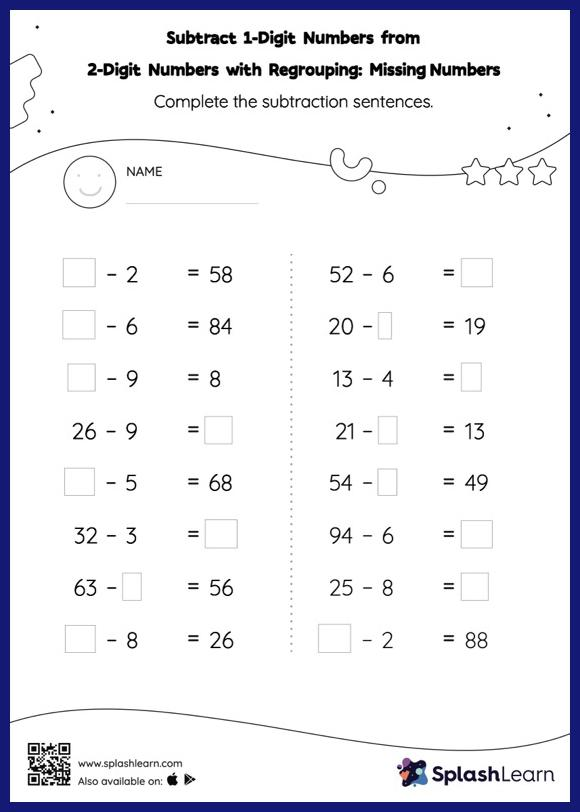# Subtract 1-Digit Numbers from 2-Digit Numbers with Regrouping: Missing Numbers Worksheet

Home > Subtract 1-Digit Numbers from 2-Digit Numbers with Regrouping: Missing NumbersIn this worksheet, students must find the missing number using the count back method or the relationship between addition and subtraction. To solve subtract 1-digit numbers from 2-digit numbers with regrouping worksheet, they must also regroup the numbers. This worksheet is about practicing with the horizontal format in which numbers are written side by side. To develop flexibility with numbers and operations, students need to have enough practice in this format and not just rely on the vertical/column method.